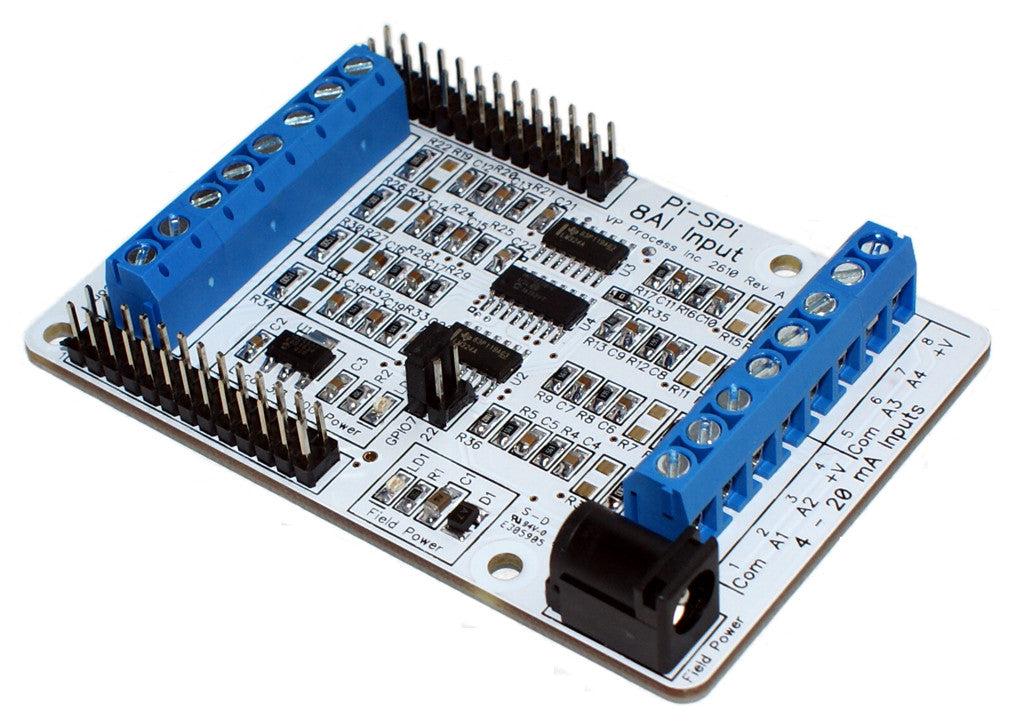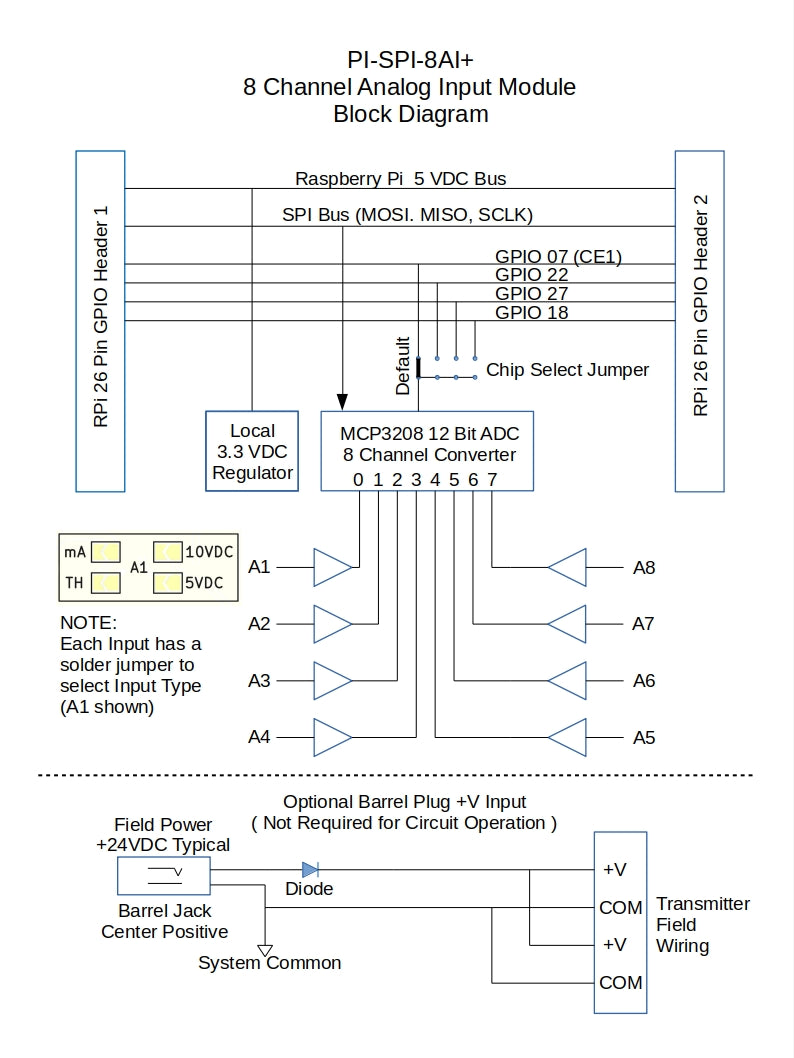# PI-SPI-8AI Python Code Samples

The PI-SPi-8AI is an 8 channel Analog Input Module that communicates with the Raspberry Pi via the SPI bus.This module has 2 chip selects:

Default Chip Select is GPIO7
Alternate Chip Select is GPIO22

The chip select operation during SPI communication is handled directly by the libwidgetlords library and kernel.

The PI-SPI-8AI has now been replaced with the PI-SPI-8AI+ which has 4 chip selects.Both modules operate exactly the same and are compatible with previous versions of the libwidgetlords libraries.

To run the sample Python code examples, please install the Widgetlords libwidgetlords version 2.0 libraries as explained in:

Getting Started with PI-SPI Libraries

NOTE: All of the sample code examples are for Python 3, and are for using 2 chip selects. The chip selects for both modules are:

Chip Select on -8AI GPIO7 = Chip Select CE1 on -8AI+
Chip Select on -8AI 22 = Chip Select 22 on -8AI+

Please ensure you have the dtoverlay set correctly in the /boot/config.txt file:

`dtoverlay=pi-spi`

In this code example:

1. We import the Widgetlords library
2. Initialize the library
3. Read a single Channel ( Channel 0 is Input A1 on the PI-SPI-8AI Module)
4. Print reading to the shell window

`from time import sleepfrom widgetlords.pi_spi import *init()inputs = Mod8AI()while True:    print(inputs.read_single(0))    sleep(0.5)`

Every Pi-SPI module has a dedicated GPIO pin for it's SPI Chip Select and an Alternate chip select to allow two modules of the same type to be used together.

This code example shows how to enable the alternate chip select. NOTE: this works for all following code examples as well!

Each module type has the Chip Select defaulted to it's unique GPIO Pin as follows:

PI-SPI-8AI = GPIO7
PI-SPI-8KO = GPIO8
PI-SPI-8DI = GPIO17
PI-SPI-2AO = GPIO4

For each module, the alternate Chip Select is GPIO22

The module initialization line for the default Chip Select is

`inputs = Mod8AI()`

OR

`inputs = Mod8AI(False)`

To use the alternate chip select:

`inputs = Mod8AI(True)`

Here is the complete sample code example using the alternate chip select

```from time import sleepfrom widgetlords.pi_spi import *init()inputs = Mod8AI(True)while True:    print(inputs.read_single(0))    sleep(0.5) ```

#### Sample 3 - Multiple Channel A/D Count Readings

This reads multiple channels 0 thru 7 (A1 thru A8) on the module and prints them to the shell window.

`from time import sleepfrom widgetlords.pi_spi import *init()inputs = Mod8AI()while True:    A1 = inputs.read_single(0)    A2 = inputs.read_single(1)    A3 = inputs.read_single(2)    A4 = inputs.read_single(3)    A5 = inputs.read_single(4)    A6 = inputs.read_single(5)    A7 = inputs.read_single(6)    A8 = inputs.read_single(7)    print(A1)    print(A2)    print(A3)    print(A4)    print(A5)    print(A6)    print(A7)    print(A8)    print("")        sleep(0.5)`

#### Sample 4 - Format A/D Reading to mA or VDC

Instead of reading just A/D counts, it may be more meaningful to display the actual reading in mA, Voltage or Temperature. By adding one more library feature, the format function is available for the user:

where:

zero_ad_counts = A/D counts to represent the Zero Value
span_ad_counts = A/D counts to represent the Span Value
zero_value = Zero Value ie: 0 mA or 4 mA
span_value = Span Value ie: 20 mA

Here is the sample code to read channel 0 (A1) in mA:
(Standard Python number formatting to limit decimal places to 2 places)

`from time import sleepfrom widgetlords.pi_spi import *from widgetlords import *init()inputs = Mod8AI()while True:    A1 = inputs.read_single(0)    print("Input 1 = %4d AD Counts, %0.2f mA" % (A1, counts_to_value(A1, 745, 3723, 4, 20 )))    print("")    sleep(0.5)`

Here is the sample code to read channel 4 (A5) in VDC:
(Standard Python number formatting to limit decimal places to 2 places)
NOTE: Range is from 0 VDC to 6.6 VDC

`from time import sleepfrom widgetlords.pi_spi import *from widgetlords import *init()inputs = Mod8AI()while True:    A5 = inputs.read_single(4)    print("Input 5 = %4d AD Counts, %0.2f VDC" % (A5, counts_to_value(A5, 0, 4095, 0, 6.6 )))    print("")    sleep(0.5)`

#### Sample 5 - Format A/D Reading to Temperature Using Steinhartt-Hart Equation

The PI-SPI-8AI-TEMP module has 8 channels of Thermistor inputs for 8 channels of temperature measurement. Each channel fo the PI-SPI-8AI can be configured as either 4-20 mA, 0 to 6.6 VDC or Thermistor Input.

Here is the format to convert A/D counts to Degree C using the Steinhart-Hart Equation:

where:

Temp = the formatted reading as Deg C
r_temp = the Thermistor Ohms at Room Temperature (25 Deg.C)
beta = the Beat value of the Thermistor being used
max_ad_counts = the maximum ad counts ie: 4095 for a 12 bit A/D converter

In our products, we use a Cantherm thermistor with a 10K Ohm at 25 Deg C, 3380 Beta and our load resistor is 10K Ohms (fixed value)

Here is the sample code to convert channel 0 (A1) to a Temperature reading:

`from time import sleepfrom widgetlords.pi_spi import *from widgetlords import *init()inputs = Mod8AI()while True:    A1 = inputs.read_single(0)    Temp = steinhart_hart(10000, 3380, 4095, A1)    print(Temp)    print("")    sleep(0.5)`

#### Sample 6 - PI-SPI-8AI-8MA Sample Code (8 Channels 4-20 mA)

`from time import sleepfrom widgetlords.pi_spi import *from widgetlords import *init()inputs = Mod8AI()while True:    A1 = inputs.read_single(0)    A2 = inputs.read_single(1)    A3 = inputs.read_single(2)    A4 = inputs.read_single(3)    A5 = inputs.read_single(4)    A6 = inputs.read_single(5)    A7 = inputs.read_single(6)    A8 = inputs.read_single(7)    print("Input 1 = %4d AD Counts, %0.2f mA" % (A1, counts_to_value(A1, 745, 3723, 4, 20 )))    print("Input 2 = %4d AD Counts, %0.2f mA" % (A2, counts_to_value(A2, 745, 3723, 4, 20 )))    print("Input 3 = %4d AD Counts, %0.2f mA" % (A3, counts_to_value(A3, 745, 3723, 4, 20 )))    print("Input 4 = %4d AD Counts, %0.2f mA" % (A4, counts_to_value(A4, 745, 3723, 4, 20 )))    print("Input 5 = %4d AD Counts, %0.2f mA" % (A5, counts_to_value(A5, 745, 3723, 4, 20 )))    print("Input 6 = %4d AD Counts, %0.2f mA" % (A6, counts_to_value(A6, 745, 3723, 4, 20 )))    print("Input 7 = %4d AD Counts, %0.2f mA" % (A7, counts_to_value(A7, 745, 3723, 4, 20 )))    print("Input 8 = %4d AD Counts, %0.2f mA" % (A8, counts_to_value(A8, 745, 3723, 4, 20 )))     print("")     sleep(0.5)  `

#### Sample 7 - PI-SPI-8AI-4MA-4VDC Sample Code (4 Channels 4-20 mA, 4 Channels 0 to 5 VDC)

`from time import sleepfrom widgetlords.pi_spi import *from widgetlords import *init()inputs = Mod8AI()while True:    A1 = inputs.read_single(0)    A2 = inputs.read_single(1)    A3 = inputs.read_single(2)    A4 = inputs.read_single(3)    A5 = inputs.read_single(4)    A6 = inputs.read_single(5)    A7 = inputs.read_single(6)    A8 = inputs.read_single(7)    print("Input 1 = %4d AD Counts, %0.2f mA" % (A1, counts_to_value(A1, 745, 3723, 4, 20 )))    print("Input 2 = %4d AD Counts, %0.2f mA" % (A2, counts_to_value(A2, 745, 3723, 4, 20 )))    print("Input 3 = %4d AD Counts, %0.2f mA" % (A3, counts_to_value(A3, 745, 3723, 4, 20 )))    print("Input 4 = %4d AD Counts, %0.2f mA" % (A4, counts_to_value(A4, 745, 3723, 4, 20 )))        print("")        print("Input 5 = %4d AD Counts, %0.2f VDC" % (A5, counts_to_value(A5, 0, 4095, 0, 6.6 )))    print("Input 6 = %4d AD Counts, %0.2f VDC" % (A6, counts_to_value(A6, 0, 4095, 0, 6.6 )))    print("Input 7 = %4d AD Counts, %0.2f VDC" % (A7, counts_to_value(A7, 0, 4095, 0, 6.6 )))    print("Input 8 = %4d AD Counts, %0.2f VDC" % (A8, counts_to_value(A8, 0, 4095, 0, 6.6 )))    print("")        sleep(0.5)`

#### Sample 8 - PI-SPI-8AI-TEMP Sample Code (8 Channels Thermistor Input)

`from time import sleepfrom widgetlords.pi_spi import *from widgetlords import *init()inputs = Mod8AI()while True:    A1 = inputs.read_single(0)    A2 = inputs.read_single(1)    A3 = inputs.read_single(2)    A4 = inputs.read_single(3)    A5 = inputs.read_single(4)    A6 = inputs.read_single(5)    A7 = inputs.read_single(6)    A8 = inputs.read_single(7)     Temp1 = steinhart_hart(10000, 3380, 4095, A1)     Temp2 = steinhart_hart(10000, 3380, 4095, A2)     Temp3 = steinhart_hart(10000, 3380, 4095, A3)     Temp4 = steinhart_hart(10000, 3380, 4095, A4)     Temp5 = steinhart_hart(10000, 3380, 4095, A5)     Temp6 = steinhart_hart(10000, 3380, 4095, A6)     Temp7 = steinhart_hart(10000, 3380, 4095, A7)     Temp8 = steinhart_hart(10000, 3380, 4095, A8)     if(A1>4050):# Test to see fo Sensor is present        print("Sensor Not present")    else:        print("Temp1 = %0.1f Deg C" % Temp1)     sleep(0.5)`

Here is the block diagram of the PI-SPI-8AI+